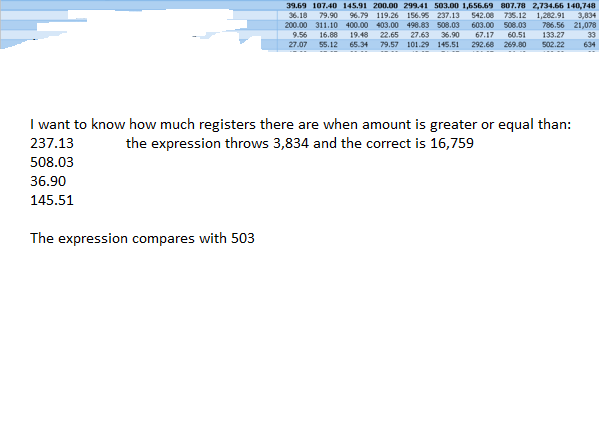# New to QlikView

Discussion board where members can get started with QlikView.

Announcements
BARC’s The BI Survey 19 makes it official. BI users love Qlik. GET REPORT
HighlightedNot applicable

## Set Analysis Greater than (fractile)

Hello,

I am trying to count how much registers there are when an amount is greater or equal to the fractile of that group.

I have the next expression, it works but it responses with the total fractile and not with the fractile of the group.

if(P_95_Fr>=P_95,count({<AMOUNT={">=\$(=fractile(AMOUNT,0.95))"}>}ACCOUNT),0)Tags (2)
6 RepliesMVP

## Re: Set Analysis Greater than (fractile)

Set expression as well as your dollar sign expansion is evaluated once per chart, not per dimension value.

Try maybe something like

=Count( Aggr( If( AMOUNT >= Fractile(TOTAL<GROUP> AMOUNT,0.95), AMOUNT), GROUP, AMOUNT))

where you need to replace GROUP with your group dimension and maybe adapt the aggr() dimensions to your chart dimension list.Not applicable

## Re: Set Analysis Greater than (fractile)

Thank you for the idea!

I changed the logic of the expression but I continue with the same result, it compare with the total and not with the fractile of the dimension.

=Aggr(count({<AMOUNT={">=\$(=fractile(TOTAL <FIELD> AMOUNT,0.95))"}>}FIELD2),FIELD)MVP

## Re: Set Analysis Greater than (fractile)

yes, as said, set analysis is not the way to go here.

Have you also tried my suggested expression?Not applicable

## Re: Set Analysis Greater than (fractile)

Yes I tried it. Only I changed the expression based on your suggestion. I didn't change the dimension because I don't understand what you want to mean in "and maybe adapt the aggr() dimensions to your chart dimension list."

With this, the result has changed but not it is the correct.MVP

## Re: Set Analysis Greater than (fractile)

Could you upload a small sample QVW and your requested results (for example in an excel file)?Not applicable